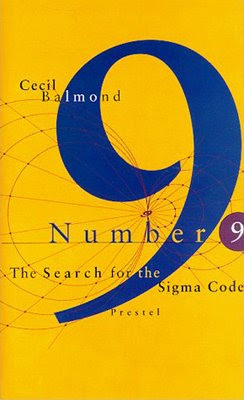## Tuesday, 2 June 2009

### Balmond's Sigma CodeThe Search for the Sigma Code has a dense plot and relies on another imaginary narrator (not a monk this time, but) a boy called Enjil. If you have ever wondered why any prime number is greater than three will, when raised to the sixth power, leave a remainder of one when divided by nine, you will be at home with this book. -- Vanessa Thorpe, The Independent On Sunday - 13 September 1998

You get the sigma code of a given number n like this:
1. Calculate the sum s of all digits of n.
2. If s is smaller then 10, s is the sigma value.
With this definition it is possible to calculate the sigma values of number sequences. The result of some simple sequences are beautiful patterns:This diagrams you obtain from the Fibonacci and the Pell sequence by using (and modifing) the following script: SigmaCode.zip